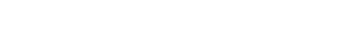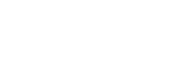Function

Resistors restrict the flow of electric current, for example a resistor is placed in series with a light-emitting diode (LED) to limit the current passing through the LED.Connecting and soldering

Resistors may be connected either way round because they are not polarized (no negative or positive). They are not damaged by heat when soldering.

Resistor values – the resistor colour code

Resistance is measured in ohms, the symbol for ohm is an omega .
1 is quite small so resistor values are often given in k and M .
1 k = 1000     1 M = 1000000 .Resistor values are normally shown using coloured bands.
Each colour represents a number as shown in the table.

Most resistors have 4 bands:

• The first band gives the first digit.
• The second band gives the second digit.
• The third band indicates the number of zeros.
• The fourth band is used to shows the tolerance (precision) of the resistor. (Gold is 5%, Silver is 10% and None is 20%)This resistor has red (2), violet (7), yellow (4 zeros) and gold bands.
So its value is 270000  ± 5%= 270 k ± 5%.
On circuit diagrams the is usually omitted and the value is written 270K.

Cite this article as: William Anderson (Schoolworkhelper Editorial Team), "Resistors: Function & Color Code," in SchoolWorkHelper, 2019, https://schoolworkhelper.net/resistors-function-color-code/.